# Using funnel charts for monitoring rates

For a recent project of mine I was exploring arrest rates at small units of analysis (street midpoints and intersections) for one of the collaborations we have at the Finn Institute.

One of the graphs I was interested in making was a funnel plot of the arrest rates on the Y axis and the total number of stops on the X axis. You can then draw confidence bands around a particular percentage (typically the overall rate) to see if any observations have oddly high or low rates. This procedure is described in Funnel plots for comparing institutional performance (Spiegelhalter, 2005), PDF Here. Funnel charts are more common in meta-analysis, but I hope to illustrate their utility here in monitoring rates with different denominators.

For an illustration I will make a funnel plot of the homicide rates per 100,000 given by the police agencies in New York and Pennsylvania in 2012 (available via the UCR data tool). Here is the data and code available to replicate the results in this blog post in a zip file. If you have the `SPSSINC GETURI DATA` add-on you can start just by calling (otherwise you have to download the data to follow along).

``````SPSSINC GETURI DATA
URI="https://dl.dropboxusercontent.com/u/3385251/NY_PA_crimerates_2012.sav"
FILETYPE=SAV DATASET=NY_PA.``````

I start at the point in the code where the data is already loaded, and I generate a scatterplot for `Population` and `Murder_and_nonnegligent_manslaughter_rate`. I eliminate agencies that did not report a full 12 months or had missing data for homicides and/or the population, and this results in around 360 homicide rates for populations above 10,000 and up nearly 10 million (in New York City). The labels for SPSS graphs inherit the format for the original data, so I make the homicide rates `F2.0` to avoid decimal places in the axis tick mark labels.

``````FORMATS Murder_and_nonnegligent_manslaughter_rate (F2.0).
GGRAPH
/GRAPHDATASET NAME="graphdataset" VARIABLES=Population Murder_and_nonnegligent_manslaughter_rate
/GRAPHSPEC SOURCE=INLINE.
BEGIN GPL
SOURCE: s=userSource(id("graphdataset"))
DATA: Population=col(source(s), name("Population"))
DATA: Murder_and_nonnegligent_manslaughter_rate=col(source(s),
name("Murder_and_nonnegligent_manslaughter_rate"))
GUIDE: axis(dim(1), label("Population"))
GUIDE: axis(dim(2), label("Murder Rate per 100,000"))
SCALE: log(dim(1))
ELEMENT: point(position(Population*Murder_and_nonnegligent_manslaughter_rate))
END GPL.``````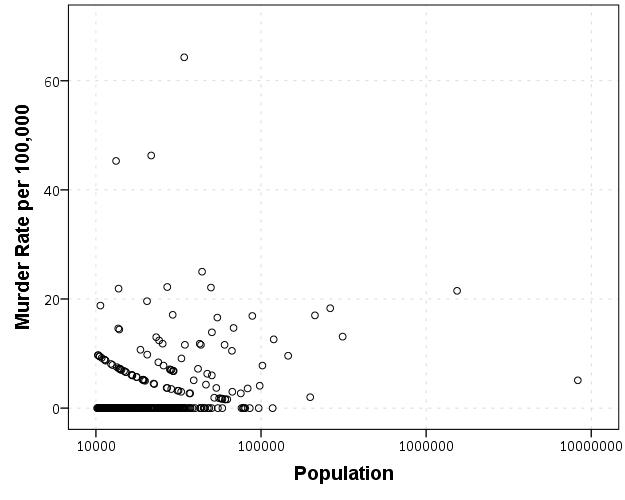So we can see that the bulk of the institutions have very low murder rates, the overall rate is just shy of 6 murders per 100,000 in this sample. But there are quite a few locations that appear to be high outliers. We are talking about proportions as small as `0.00006` to `0.00065` though, so are some of the high murder rate locations really that surprising? Lets see by adding an estimate of the error if the individual rates were drawn from the same distribution as the overall rate.

Now to make the funnel chart we need to estimate the confidence interval for a rate of `6/100,000` for population sizes between 10,000 and 10 million to add to our chart. We can add these interpolated bounds directly into our SPSS dataset. So to estimate the lines what I do is use the `AGGREGATE` command to interpolate population step sizes for the min and max population in the sample (and calculate the total homicide rate). Here I interpolate the population steps on the log scale, as the X axis in the chart uses a log scale.

``````*Aggregate proportions and max/min counts.
COMPUTE Id = \$casenum - 1.
/BREAK
/MaxPop = MAX(Population)
/MinPop = MIN(Population)
/AllHom = SUM(Murder_and_nonnegligent_Manslaughter)
/TotalPop = SUM(Population)
/TotalObs = MAX(Id).
*Overall homicide rate per 100,000.
COMPUTE HomRate = (AllHom/TotalPop)*100000.
*Now interpolating bounds for the population.
COMPUTE #Step = (LG10(MaxPop) - LG10(MinPop))/TotalObs.
COMPUTE N = 10**(LG10(MinPop) + Id*#Step).``````

So if you see the variable `N` in the dataset now you will see that the highest value is equal to the population in New York City at over 8 million. SPSS does not have an inverse function for the binomial distribution, but it does have one for the beta distribution. Wikipedia lists how the Clopper-Pearson binomial confidence intervals can be rewritten in terms of the beta distribution. I use this equality here to generate 99% confidence intervals for the population sizes in the `N` variable for the overall homicide rate, `HomeRate` (remembering to convert rates per 100,000 back to proportions).

``````*Now calculating bands based on statewide homicide rates.
*Exact bounds based on inverse beta.
*http://en.wikipedia.org/wiki/Binomial_proportion_confidence_interval#Clopper-Pearson_interval.
COMPUTE #Alph = 0.01.
COMPUTE #Suc = (HomRate*N)/100000.
COMPUTE LowB = IDF.BETA(#Alph/2,#Suc,N-#Suc+1)*100000.
COMPUTE HighB = IDF.BETA(1-#Alph/2,#Suc+1,N-#Suc)*100000.
EXECUTE.``````

Now we can superimpose `LowB`, `HighB`, and `HomRate` on the same graph as the previous scatterplot to give a sense of the uncertainty in the estimates. Here I also change the size of the graph using `PAGE` statements. I color the error bounds as light grey lines, and the overall homicide rate as a red line, and then superimpose the homicide rates for each agency on top of them.

``````*Now can make the plot with the error bounds.
FORMATS LowB HighB HomRate (F2.0) N (F8.0).
GGRAPH
/GRAPHDATASET NAME="graphdataset" VARIABLES=Population Murder_and_nonnegligent_manslaughter_rate
LowB HighB HomRate N
/GRAPHSPEC SOURCE=INLINE.
BEGIN GPL
PAGE: begin(scale(600px,800px))
SOURCE: s=userSource(id("graphdataset"))
DATA: Population=col(source(s), name("Population"))
DATA: Murder_and_nonnegligent_manslaughter_rate=col(source(s),
name("Murder_and_nonnegligent_manslaughter_rate"))
DATA: LowB=col(source(s), name("LowB"))
DATA: HighB=col(source(s), name("HighB"))
DATA: HomRate=col(source(s), name("HomRate"))
DATA: N=col(source(s), name("N"))
GUIDE: axis(dim(1), label("Population"))
GUIDE: axis(dim(2), label("Murder Rate per 100,000"), delta(2))
SCALE: log(dim(1))
SCALE: linear(dim(2), min(2), max(64))
ELEMENT: line(position(N*HomRate), color(color.red), size(size."2"))
ELEMENT: line(position(N*LowB), color(color.grey))
ELEMENT: line(position(N*HighB), color(color.grey))
ELEMENT: point(position(Population*Murder_and_nonnegligent_manslaughter_rate), size(size."5"),
transparency.exterior(transparency."0.5"))
PAGE: end()
END GPL.``````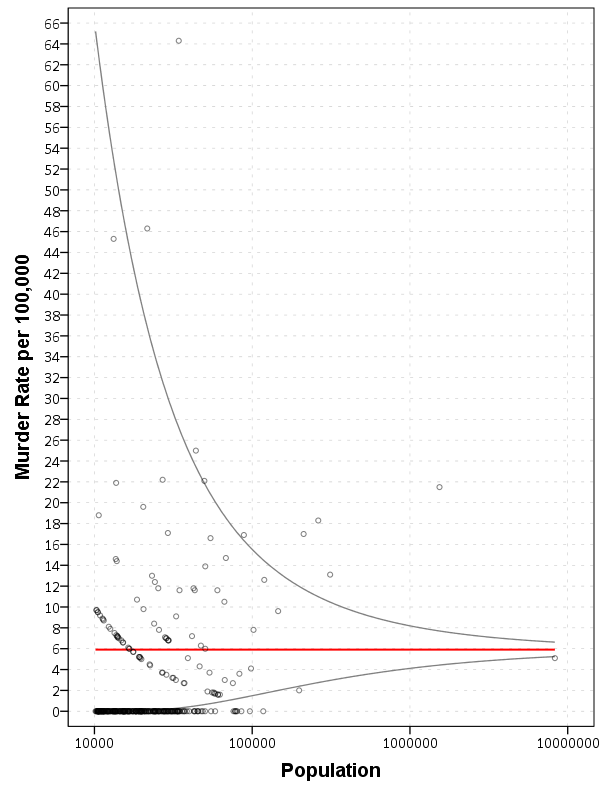So I hope you see where the name funnel chart comes from now! This chart gives us an idea of the variability we would expect if random data were drawn with a rate of 6/100000 for population sizes between 10,000 and 100,000 is quite large, and most of the agencies with high homicide rates are still below the upper bound. Even with a population of 100,000, the 99% confidence interval covers rates between 2 and almost 16 per 100,000 – which translates directly to 2 and 16 homicides in a jurisdiction of that size.

Here I add labels to the chart for locations above the interval and below the interval (but at least have one homicide). I add the total number of homicides in that jurisdiction in parenthesis to the label as well.

``````*Now adding in labels.
COMPUTE #Alph = 0.01.
COMPUTE #Suc = (HomRate*Population)/100000.
COMPUTE LowA = IDF.BETA(#Alph/2,#Suc,Population-#Suc+1)*100000.
COMPUTE HighA = IDF.BETA(1-#Alph/2,#Suc+1,Population-#Suc)*100000.
EXECUTE.

STRING HighLab (A50).
IF Murder_and_nonnegligent_manslaughter_rate > HighA
HighLab = CONCAT(RTRIM(REPLACE(Agency,"Police Dept",""))," (",LTRIM(STRING(Murder_and_nonnegligent_Manslaughter,F3.0)),")").
IF Murder_and_nonnegligent_manslaughter_rate  0
HighLab = CONCAT(RTRIM(REPLACE(Agency,"Police Dept",""))," (",LTRIM(STRING(Murder_and_nonnegligent_Manslaughter,F3.0)),")").
EXECUTE.``````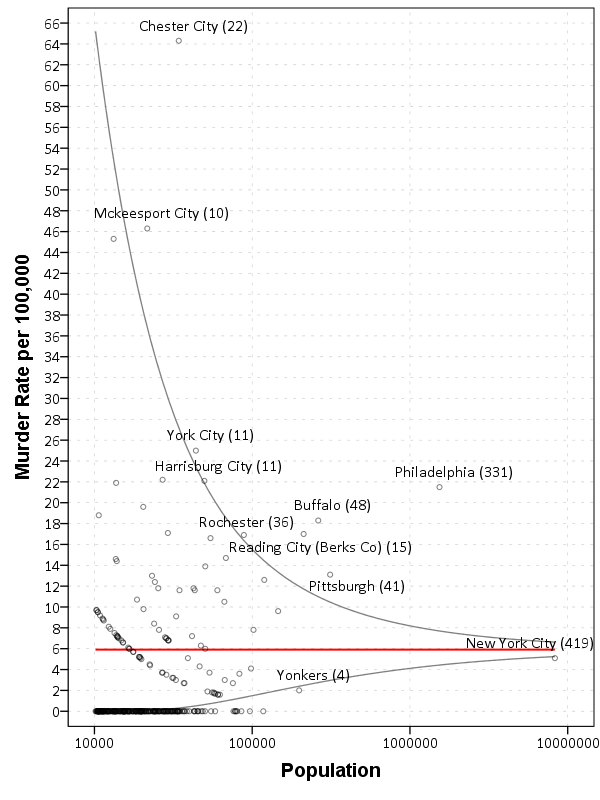The labels get a little busy, but we can see that save for Buffalo and Rochester, all of the jurisdictions with higher than expected homicide rates are in Pennsylvania, with Philadelphia being the most egregious outlier. New York City and Yonkers are actually lower than the confidence interval, although quite close (along with a set of cities with zero homicides in populations between mainly the lower bound of 10,000 to 100,000). Chester city and Mckeesport are high locations as well, but we can see from the labels they only have 22 and 10 homicides respectively. The point to the left of Mckeesport is Coatesville, which ends up having only 6 homicides.

Where I consider such charts useful are to identify outliers (such as Philadelphia and Chester City). Depending on the nature of the study will depend on what this information could be useful for. For a reporting agency like the FBI they may be interested in seeing if Chester City is a reporting anomaly, or the NIJ/DOJ may be interested in funnelling resources to these high homicide rate locations. The same is true for a police department monitoring rates of say contraband recovery at particular locations, or uses of force per officer incident.

For a researcher they may be interested in other causal factors that could cause a high or low rate, as this might give insight into the mechanisms that increase or decrease homicides (for this particular chart). Evaluating against the overall homicide rate for the sample is an ad-hoc decision (it appears in this chart that PA and NY may plausibly have distinct homicide rate values, with PA being higher) but it at least gives the analyst an idea of the variability when evaluating rates.

# Understanding Uncertainty – crime counts and the Poisson distribution

A regular occurrence for me when I was a crime analyst went along the lines of, "There was a noteworthy crime event in the media, can you provide some related analysis". Most of the time this followed one or multiple noteworthy crimes that caught the public’s attention, which could range from a series of thefts from vehicles over a month, the same gas station being robbed on consecutive days, or a single murder.

Any single violent crime is awful, and this is not meant to deny that. But often single noteworthy events are often misconstrued as crime waves, or general notions that the neighborhood is in decline or the city is a more dangerous place now than it ever was. The media is intentionally hyperbole, so it is not an effective gauge whether or not crime is really increasing or decreasing. Here I will show an example of using the Poisson distribution to show whether or not a recent spree of crimes is more than you would expect by chance.

So lets say that over the course of 20 years, the mean number of homicides in a jurisdiction is 2. Lets also say that in the year so far, we have 5 homicides. Ignoring that the year has not concluded, what is the probability of observing 5 or more homicides? Assuming the number of homicides is a Poisson distributed random variable (often not too unreasonable for low counts over long time periods) the probability is 5.7%. Small, but not totally improbable. To calculate this probability it is just 1 minus the cumulative distribution function for a Poisson distribution with the given mean. This can be calculated easily in R by using `ppois`, i.e. `1 - ppois(5-1,2)` (just replace `5` with your observed count and `2` with your mean). Note that I subtract 1 from 5, otherwise it would be testing the probability of over 5 instead of 5 or more. For those analysts using Excel, the formula is `=1 - POISSON.DIST(5-1,2,TRUE)`. For SPSS it would be `COMPUTE Prob = 1 - CDF.POISSON(5-1,2)`.

The reason for making these calculations is specifically to understand chance variations given the numbers historically. For the crime analyst it is necessary to avoid chasing noise. For the public it is necessary to understand the context of the current events in light of historical data. For another example, say that the average number of robberies in a month is 15, what is the probability of observing 21 or more robberies? It is 8%, so basically you would expect this high of a number to happen at least one time every year (i.e. 0.08*12~1). Without any other information, there is little reason for a crime analyst to spend extra time examining 21 robberies in a month based on the total number of events alone. Ditto for the public there is little reason to be alarmed by that many robberies in a month given the historical data. (The analyst may want to examine the robberies for other reasons, but there is no reason to be fooled into thinking there is an unexpected increase.)

Here I’ve ignored some complications for the sake of simplicity in the analysis. One is that crime may not be Poisson distributed, but may be under or over-dispersed. In the case of over-dispersion (which seems to happen more often with crime data) the series likely has a higher number of 0’s and then high bursts of activity. In this case you would expect the higher bursts more often than you would with the Poisson distribution. For under-dispersed Poisson data, the variance is smaller than the mean, and so higher bursts of activity are less likely. These are fairly simple to check (at least to see if they are grossly violated), either see if the mean approximately equals the variance, or draw a histogram and superimpose a density estimate for the Poisson distribution. This also ignores seasonal fluctuations in crime (e.g. more burglaries occur in the summer than in the winter).

Even if you do not like making the Poisson assumption a very simple analysis to conduct is to plot the time series of the event over a long period. The rarer the crime the larger aggregation and time series you might need, but this is pretty straightforward to conduct with a SQL query and whatever program you use to conduct analysis. If it is for UCR crime counts, you can try going onto the UCR data tool to see if your jurisdiction has historical annual data going back to 1985. My experience is the vast majority of crime waves depicted in the media are simply chance fluctuations, clearly visible as such just by inspecting the time series plot. Similarly such a plot will show if there is an increase or a decrease compared to historical numbers. Another simple analysis is to take the current numbers and rank them against, say the prior 50 to 100 values in the series. If it is abnormal it should be the the highest or very near the highest in those prior values.

Another complication I have ignored is that of multiple testing. When one is constantly observing a series, even a rare event is likely to happen over a long period of observation. So lets say that in your jurisdiction on average there are 3 domestic assaults in a week, and one week you observe 9. The probability of observing 9 or more is 0.003, but over a whole year the probability of this happening at least once is around 18% (i.e. `1 - ppois(8,3)^52` in R code). Over the course of 10 years (around 520 weeks) we would expect around 2 weeks to have 9 or more domestic assaults (i.e. `520*(1-ppois(8,3))`). (This probability goes up higher if we consider sliding windows, e.g. 9 or more domestic assaults in any 7 day span, instead of just over different weeks.) These statistics make the assumption that events are independent (likely not true in practice) but I rather make that false assumption to get a sense of the probability then rely on gut feelings or opinions based on the notoriety of the recent crime(s).

The title for this blog post is taken from David Spiegelhalter’s site Understanding Uncertainty, and that link provides a synonymous example with the recent cluster of plane crashes.

# Odds Ratios NEED To Be Graphed On Log Scales

Andrew Gelman blogged the other day about an example of Odds Ratios being plotted on a linear scale. I have seen this mistake a couple of times, so I figured it would be worth the time to further elaborate on.

Reported odds ratios are almost invariably from the output of a generalized linear regression model (e.g. logistic, poisson). Graphing the associated exponentiated coefficients and their standard errors (or confidence intervals) is certainly a reasonable thing to want to do – but unless someone wants to be misleading they need to be on a log scale. When the coefficients (and the associated intervals) are exponeniated they are no longer symmetric on a linear scale.

To illustrate a few nefarious examples, lets pretend our software spit out a series of regression coefficients. The table shows the original coefficients on the log odds scale, and the subsequent exponentiated coefficients +- 2 Standard Errors.

``````Est.  Point  S.E. Exp(Point) Exp(-2*S.E.) Exp(+2*S.E.)
1   -0.7   0.1    0.5        0.4            0.6
2    0.7   0.1    2.0        1.6            2.5
3    0.2   0.1    1.2        1.0            1.5
4    0.1   0.8    1.1        0.2            5.5
5   -0.3   0.9    0.7        0.1            4.5``````

Now, to start lets graph the exponentiated estimates (the odds ratios) for estimates `1` and `2` and their standard errors on an arithmetic scale, and see what happens.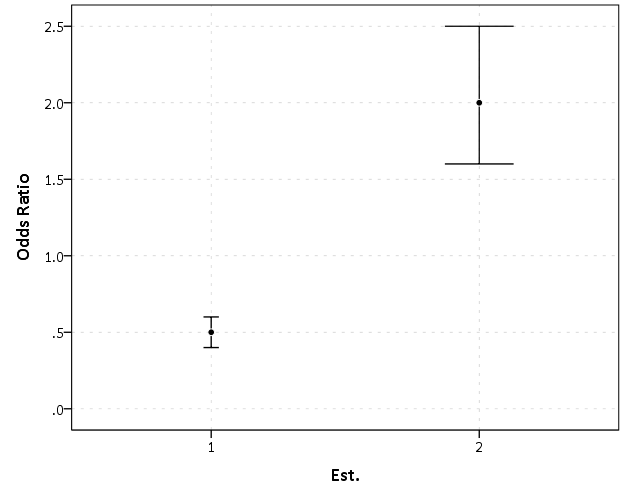This graph would give the impression that `2` is both a larger effect and has a wider variance than effect `1`. Now lets look at the same chart on a log scale.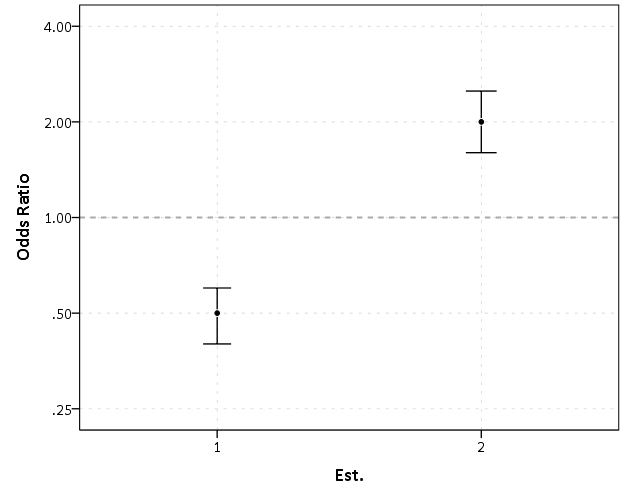By construction effects `1` and `2` are exactly the same (this is clear on the original log odds scale before the coefficients were exponentiated). Changes in the ratio of the odds can not go below zero, and a change from an odds ratio between 0.5 and 0.4 is the same relative change as that between 2.0 and 2.5. On the linear scale though the former is a difference of 0.1, and the latter a difference of 0.5.

Such visual discrepancies get larger the further towards zero you go, and as what goes in the denominator and what goes in the numerator is arbitrary, displaying these values on a linear scale is very misleading. Consider a different example: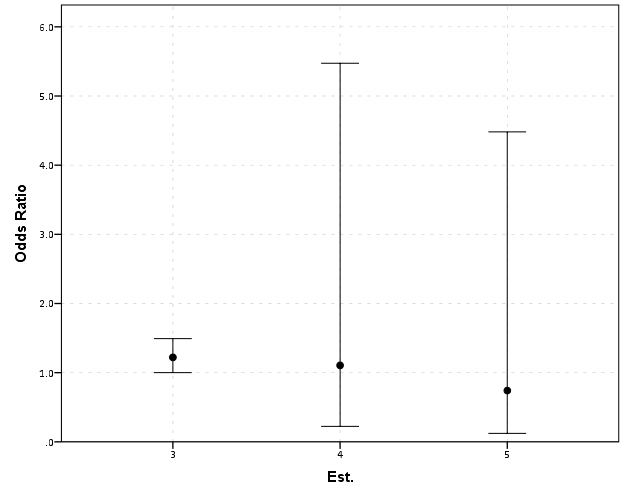Well, what would we gather from this? Estimates `4` and `5` both have a wide variance, and the majority of their error bars are both above 1. This is an incorrect interpretation though, as the point estimate of `5` is below 1, and more of its error bar is on the below 1 side.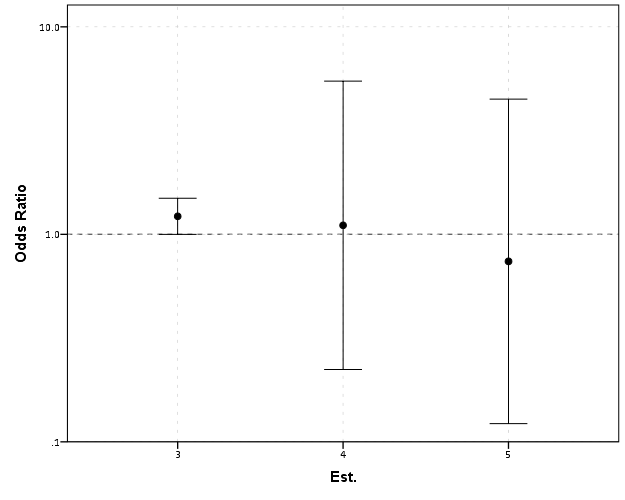Looking up some more examples online this may be a problem more often than I thought (doing a google image search for “plot odds ratios” turns up plenty of examples to support my position). I even see some examples of forest plots of odds ratios fail to do this. An oft critique of log scales is that they are harder to understand. Even if I acquiesce that this is true, plotting odds ratios on a linear scale is misleading and should never be done.

To make a set of charts in SPSS with log scales for your particular data you can simply enter in the model estimates using `DATA LIST` and then use `GGRAPH` to make the plot. In particular for `GGRAPH` see the `SCALE` lines to set the base of the logarithms. Example below:

``````*Can input your own data.
DATA LIST FREE / Id  (A10) PointEst  SEPoint Exp_Point CIExp_L CIExp_H.
BEGIN DATA
1   -0.7   0.1    0.5        0.4            0.6
2    0.7   0.1    2.0        1.6            2.5
3    0.2   0.1    1.2        1.0            1.5
4    0.1   0.8    1.1        0.2            5.5
5   -0.3   0.9    0.7        0.1            4.5
END DATA.
DATASET NAME OddsRat.

*Graph of Confidence intervals on log scale.
FORMATS Exp_Point CIExp_L CIExp_H (F2.1).
GGRAPH
/GRAPHDATASET NAME="graphdataset" VARIABLES=Id Exp_Point CIExp_L CIExp_H
/GRAPHSPEC SOURCE=INLINE.
BEGIN GPL
SOURCE: s=userSource(id("graphdataset"))
DATA: Id=col(source(s), name("Id"), unit.category())
DATA: Exp_Point=col(source(s), name("Exp_Point"))
DATA: CIExp_L=col(source(s), name("CIExp_L"))
DATA: CIExp_H=col(source(s), name("CIExp_H"))
GUIDE: axis(dim(1), label("Point Estimate and 95% Confidence Interval"))
GUIDE: axis(dim(2))
GUIDE: form.line(position(1,*), size(size."2"), color(color.darkgrey))
SCALE: log(dim(1), base(2), min(0.1), max(6))
ELEMENT: edge(position((CIExp_L+CIExp_H)*Id))
ELEMENT: point(position(Exp_Point*Id), color.interior(color.black),
color.exterior(color.white))
END GPL.``````

# Viz. weighted regression in SPSS and some discussionHere I wish to display some examples of visually weighted regression diagnostics in SPSS, along with some discussion about the goals and relationship to the greater visualization literature I feel is currently missing from the disscussion. To start, the current label of “visually weighted regression” can be attributed to Solomon Hsiang. Below are some of the related discussions (on both Solomon’s and Andrew Gelman’s blog), and a paper Solomon has currently posted on SSRN.

Also note that Felix Schonbrodt has provided example implementations in R. Also the last link is an example from the is.R() blog.

Hopefully that is near everyone (and I have not left out any discussion!)

A rundown of their motivation (although I encourage everyone to either read Solomon’s paper or the blog posts), is that regression estimates have a certain level of uncertainty. Particularly at the ends of the sample space of the independent variable observations, and especially for non-linear regression estimates, the uncertainty tends to be much greater than where we have more sample observations. The point of visually weighted regression is to deemphasize area of the plot where our uncertainty about the predicted values is greatest. This conversely draws ones eye to the area of the plot where the estimate is most certain.

I’ll discuss the grammar of these graphs a little bit, and from there it should be clear how to implement them in whatever software (as long as it supports transparency and fitting the necessary regression equations!). So even though I’m unaware of any current SAS (or whatever) examples, I’m sure they can be done.

## SPSS Implementation

First lets just generate some fake data in SPSS, fit a predicted regression line, and plot the intervals using lines.

``````
*******************************.
set seed = 10. /* sets random seed generator to make exact data reproducible */.
input program.
loop #j = 1 to 100. /*I typically use scratch variables (i.e. #var) when making loops.
compute X = RV.NORM(0,1). /*you can use the random number generators to make different types of data.
end case.
end loop.
end file.
end input program.
dataset name sim.
execute. /*note spacing is arbitrary and is intended to make code easier to read.
*******************************.

compute Y = 0.5*X + RV.NORM(0,SQRT(0.5)).
exe.

REGRESSION
/MISSING LISTWISE
/STATISTICS COEFF OUTS R ANOVA
/CRITERIA=PIN(.05) POUT(.10) CIN(95)
/NOORIGIN
/DEPENDENT Y
/METHOD=ENTER X
/SAVE PRED MCIN.

*Can map seperate variable based on size of interval to color and transparency.

compute size = UMCI_1 - LMCI_1.
exe.
formats UMCI_1 LMCI_1 Y X size PRE_1 (F1.0).

*Simple chart with lines.
GGRAPH
/GRAPHDATASET NAME="graphdataset" VARIABLES=X Y PRE_1 LMCI_1 UMCI_1 MISSING=LISTWISE
REPORTMISSING=NO
/GRAPHSPEC SOURCE=INLINE DEFAULTTEMPLATE=yes.
BEGIN GPL
SOURCE: s=userSource(id("graphdataset"))
DATA: X=col(source(s), name("X"))
DATA: Y=col(source(s), name("Y"))
DATA: PRE_1=col(source(s), name("PRE_1"))
DATA: LMCI_1=col(source(s), name("LMCI_1"))
DATA: UMCI_1=col(source(s), name("UMCI_1"))
GUIDE: axis(dim(1), label("X"))
GUIDE: axis(dim(2), label("Y"))
SCALE: linear(dim(1), min(-3.8), max(3.8))
SCALE: linear(dim(2), min(-3), max(3))
ELEMENT: point(position(X*Y), size(size."2"))
ELEMENT: line(position(X*PRE_1), color.interior(color.red))
ELEMENT: line(position(X*LMCI_1), color.interior(color.grey))
ELEMENT: line(position(X*UMCI_1), color.interior(color.grey))
END GPL.
``````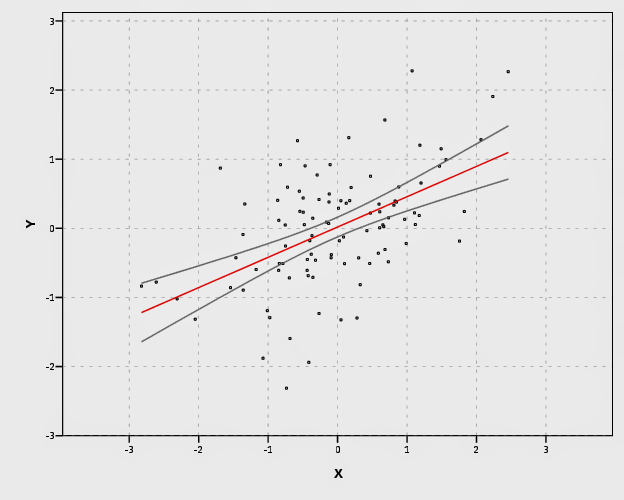In SPSS’s grammar, it is simple to plot the predicted regression line with areas of the higher confidence interval as more transparent. Here I use saved values from the regression equation, but you can also use functions within GPL (see `smooth.linear` and `region.confi.smooth`).

``````
*Using path with transparency between segments.
GGRAPH
/GRAPHDATASET NAME="graphdataset" VARIABLES=X Y PRE_1 size
/GRAPHSPEC SOURCE=INLINE.
BEGIN GPL
SOURCE: s=userSource(id("graphdataset"))
DATA: X=col(source(s), name("X"))
DATA: Y=col(source(s), name("Y"))
DATA: PRE_1=col(source(s), name("PRE_1"))
DATA: size=col(source(s), name("size"))
GUIDE: axis(dim(1), label("X"))
GUIDE: axis(dim(2), label("Y"))
GUIDE: legend(aesthetic(aesthetic.transparency.interior), null())
SCALE: linear(dim(1), min(-3.8), max(3.8))
SCALE: linear(dim(2), min(-3), max(3))
ELEMENT: point(position(X*Y), size(size."2"))
ELEMENT: line(position(X*PRE_1), color.interior(color.red), transparency.interior(size), size(size."4"))
END GPL.
``````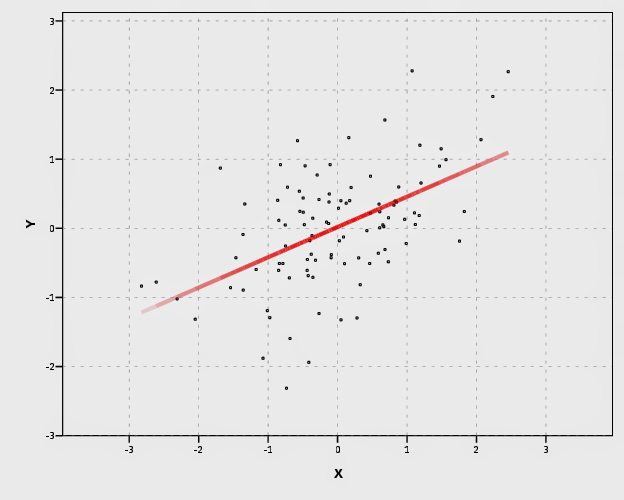It is a bit harder to make the areas semi-transparent throughout the plot. If you use an `area.difference` ELEMENT, it makes the entire area a certain amount of transparency. If you use intervals with the current data, the area will be too sparse. So what I do is make new data, sampling more densley along the x axis and make the predictions. From this we can use interval element to plot the predictions, mapping the size of the interval to transparency and color saturation.

``````
*make new cases to have a consistent sampling of x values to make the intervals.

input program.
loop #j = 1 to 500.
compute #min = -5.
compute #max = 5.
compute X = #min + (#j - 1)*(#max - #min)/500.
compute new = 1.
end case.
end loop.
end file.
end input program.
dataset name newcases.
execute.

dataset activate sim.
/file = 'newcases'.
exe.
dataset close newcases.

match files file = *
/drop UMCI_1 LMCI_1 PRE_1.

REGRESSION
/MISSING LISTWISE
/STATISTICS COEFF OUTS R ANOVA
/CRITERIA=PIN(.05) POUT(.10) CIN(95)
/NOORIGIN
/DEPENDENT Y
/METHOD=ENTER X
/SAVE PRED MCIN.

compute size = UMCI_1 - LMCI_1.
formats ALL (F1.0).

temporary.
select if new = 1.
GGRAPH
/GRAPHDATASET NAME="graphdataset" VARIABLES=X PRE_1 LMCI_1 UMCI_1 size MISSING=LISTWISE
REPORTMISSING=NO
/GRAPHSPEC SOURCE=INLINE.
BEGIN GPL
SOURCE: s=userSource(id("graphdataset"))
DATA: X=col(source(s), name("X"))
DATA: PRE_1=col(source(s), name("PRE_1"))
DATA: LMCI_1=col(source(s), name("LMCI_1"))
DATA: UMCI_1=col(source(s), name("UMCI_1"))
DATA: size=col(source(s), name("size"))
TRANS: sizen = eval(size*-1)
TRANS: sizer = eval(size*.01)
GUIDE: axis(dim(1), label("X"))
GUIDE: axis(dim(2), label("Y"))
GUIDE: legend(aesthetic(aesthetic.transparency.interior), null())
GUIDE: legend(aesthetic(aesthetic.transparency.exterior), null())
GUIDE: legend(aesthetic(aesthetic.color.saturation.exterior), null())
SCALE: linear(aesthetic(aesthetic.transparency), aestheticMinimum(transparency."0.90"), aestheticMaximum(transparency."1"))
SCALE: linear(aesthetic(aesthetic.color.saturation), aestheticMinimum(color.saturation."0"),
aestheticMaximum(color.saturation."0.01"))
SCALE: linear(dim(1), min(-5.5), max(5))
SCALE: linear(dim(2), min(-3), max(3))
ELEMENT: interval(position(region.spread.range(X*(LMCI_1 + UMCI_1))), transparency.exterior(size), color.exterior(color.red), size(size."0.005"),
color.saturation.exterior(sizen))
ELEMENT: line(position(X*PRE_1), color.interior(color.darkred), transparency.interior(sizer), size(size."5"))
END GPL.
exe.
``````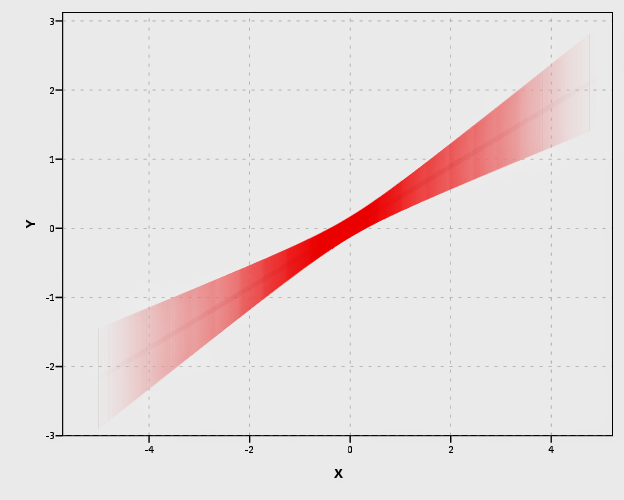Unfortunately, this creates some banding effects. Sampling fewer points makes the banding effects worse, and sampling more reduces the ability to make the plot transparent (and it is still produces banding effects). So, to produce one that looks this nice took some experimentation with how densely the new points were sampled, how aesthetics were mapped, and how wide the interval lines would be.

I tried to map a size aesthetic to the line and it works ok, you can still see some banding effects. Also note, to get the size of the line to be vertical (as oppossed to oriented in whatever direction the line is orientated) one needs to use a step line function. The `jump()` specification makes it so the vertical lines in the step chart aren’t visible. It took some trial and error to map the size to the exact interval (not sure if one can use the percent specifications to fix that trial and error). The syntax for lines though generalizes to multiple groups in SPSS easier than does the interval elements (although Solomon comments on one of Felix’s blog post that he prefers the non-interval plots with multiple groups, due to drawing difficulties and getting too busy I believe). Also FWIW it took less fussing with the density of the sample points and drawing of transparency to make the line mapped to size look nice.

``````
temporary.
select if new = 1.
GGRAPH
/GRAPHDATASET NAME="graphdataset" VARIABLES=X PRE_1 LMCI_1 UMCI_1 size MISSING=LISTWISE
REPORTMISSING=NO
/GRAPHSPEC SOURCE=INLINE.
BEGIN GPL
SOURCE: s=userSource(id("graphdataset"))
DATA: X=col(source(s), name("X"))
DATA: PRE_1=col(source(s), name("PRE_1"))
DATA: LMCI_1=col(source(s), name("LMCI_1"))
DATA: UMCI_1=col(source(s), name("UMCI_1"))
DATA: size=col(source(s), name("size"))
GUIDE: axis(dim(1), label("X"))
GUIDE: axis(dim(2), label("Y"))
GUIDE: legend(aesthetic(aesthetic.transparency.interior), null())
GUIDE: legend(aesthetic(aesthetic.transparency.exterior), null())
GUIDE: legend(aesthetic(aesthetic.size), null())
SCALE: linear(aesthetic(aesthetic.size), aestheticMinimum(size."0.16in"), aestheticMaximum(size."1.05in"))
SCALE: linear(aesthetic(aesthetic.transparency.interior), aestheticMinimum(transparency."0.5"), aestheticMaximum(transparency."1"))
SCALE: linear(dim(1), min(-5.5), max(5))
SCALE: linear(dim(2), min(-3), max(3))
ELEMENT: line(position(smooth.step.left(X*PRE_1)), jump(), color.interior(color.black), transparency.interior(size), size(size))
ELEMENT: line(position(X*PRE_1), color.interior(color.black), transparency.interior(size), size(size."3"))
END GPL.
exe.
*Add these two lines to see the actual intervals line up (without ending period).
*ELEMENT: line(position(X*LMCI_1), color.interior(color.black)).
*ELEMENT: line(position(X*UMCI_1), color.interior(color.black)).
``````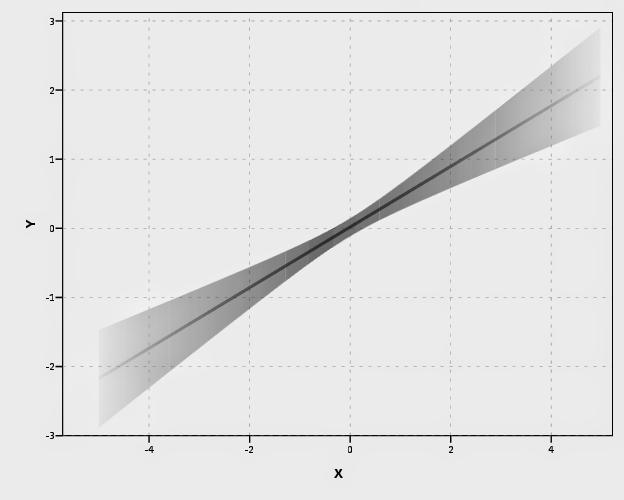Also note Solomon suggests that the saturation of the plot should be higher towards the mean of the predicted value. I don’t do that here (but have a superimposed predicted line you can see). A way to do that in SPSS would be to make multiple intervals and continually superimpose them (see Maltz and Zawitz (1998) for an applied example of something very similar). Another way may involve using color gradients for the intervals (which isn’t possible through syntax, but is through a chart template). There are other examples that I could do in SPSS (spaghetti plots of bootstrapped estimates, discrete bins for certain intervals) but I don’t provide examples of them for reasons noted below.

I will try to provide some examples of more interesting non-linear graphs in the near future (I recently made a macro to estimate restricted cubic spline bases). But this should be enough for now to show how to implement such plots in SPSS for everyone, and the necessary grammar to make the charts.

## Some notes on current discussion and implementations

My main critiques of the current implementations (and really more so the discussion) are more curmudgeonly than substantive, but hopefully it is clear enough to provide some more perspective on the work. I’ll start by saying that, it is unclear if either Solomon or Felix have read the greater visualization literature on visualizing uncertainty. While I can’t fault Felix for that so much (it is a bit much to expect a blog post to have a detailed bibliography) Solomon has at least posted a paper on SSRN. I don’t mean this as too much of a critique to Solomon, he has a good idea, and a good perspective on visualization (if you read his blog he has plenty of nice examples). But, it is more aimed at a particular set of potential alternative plots that Solomon and Felix have presented that I don’t feel are very good ideas.

Like all scientific work, we stand on the shoulders of those who come before us. While I haven’t explicitly seen visually weighted regression plots in any prior work, there are certainly many very similar examples. The most obvious would probably be the discussion of Jackson (2008), and for applied examples of this technique to very similar regression contexts are Maltz and Zawitz (1998) and Esarey and Pierce (2012) (besides Jackson (2008), there is a variety of potential other related literature cited in the discussion to Gelman’s blog posts – but this article is blatently related). There are undoubtedly many more, likely even older than the Maltz paper, and there is a treasure trove of papers about displaying error bars on this Andrew Gelman post. Besides proper attribution, this isn’t just pedantic, we should take the lessons learned from the prior literature and apply them to our current situation. There are large literatures on visualizaing uncertainty, and it is a popular enough topic that it has its own sections in cartography and visualization textbooks (MacEachren 2004; Slocum et al. 2005; Wilkinson 2005).

In particular, there is one lesson I feel should strongly reflect on the current discussion, and that is visualizing crisp lines in graphics implies the exact opposite of uncertainty to the viewers. Spiegelhalter, Pearson, and Short (2011) have an example of this, where a graphic about a tornado warning was taken a bit more to heart than it should have, and instead of people interpreting the areas of highest uncertainty in the predicted path as just that, they interpreted it as more a deterministic. There appears to be good agreement about using alpha blending (Roth, Woodruff, and Johnson 2010), and having fuzzy lines are effective ways of displaying uncertainty (MacEachren 2004; Wood et al. 2012). Thus, we have good evidence that, if the goal of the plot is to deemphasize portions of the regression that have large amounts of uncertainty in the estimates, we should not plot those estimates using discrete cut-offs. This is why I find Felix’s poll results unfortunate, in that the plot with the discrete cut-offs is voted the highest by viewers of the blog post!

So here is an example graph by Felix of the discrete bins (note this is the highest voted image in Felix’s poll!). Again to be clear, discrete bins suggests the exact opposite of uncertainty, and certainly does not deemphasize areas of the plot that have the greatest amount of uncertainty.Here is the example of the plot from Solomon that also has a similar problem with discrete bins. The colors portray uncertainty, but it is plain to see the lattice on the plot, and I don’t understand why this meets any of Solomon’s original goals. It takes ink to plot the lattice!More minor, but still enough to guide our implementations, the plots that superimpose multiple bootstrapped estimates, while they are likely ok to visualize uncertainty, the resulting spaghetti make the plots much more complex. The shaded areas maintain a much more condense and clear to understand visual, while superimposing multiple lines on the plot creates a difficult to envision distribution (here I have an example on the CV blog, and it is taken from Carr and Pickle (2009)). It may aid understanding uncertainty about the regression estimate, but it detracts from visualizing any more global trends in the regression estimate. It also fails to meet the intial goal of deemphasizing areas of the plot that are most uncertain. It accomplishes quite the opposite actually, and areas where the bootstrapped estimates have a greater variance will draw more attention because they are more dispersed on the plot.

Here is an example from Solomon’s blog of the spaghetti plots with the lines drawn heavily transparent.Solomon writes about the spaghetti plot distraction in his SSRN paper, but still presents the examples as if they are reasonable alternatives (which is strange). I would note these would be fine and dandy if visualizing the uncertainty was itself an original goal of the plot, but that isn’t the goal! To a certain extent, displaying an actual interval is countervailing to the notion of deemphasizing that area of the chart. The interval needs to command a greater area of the chart. I think Solomon has some very nice examples where the tradeoff is reasonable, with plotting the areas with larger intervals in lower color saturation (here I use transparency to the same effect). I doubt this is news to Solomon – what he writes in his SSRN paper conforms to what I’m saying as far as I can tell – I’m just confused why he presents some examples as if they are reasonable alternatives. I think it deserves reemphasis though given all the banter and implementations floating around the internet, especially with some of the alternatives Felix has presented.

I’m not sure if Solomon and Felix really appreciate though the distinction between hard lines and soft lines though after reading the blog post(s) and the SSRN paper. Of course these assertions and critiques of mine should be tested in experimental settings, but we should not ignore prior research in spite of a lack of experimental findings. I don’t want these critiques to be viewed too harshly though, and I hope Solomon and Felix take them to heart (either in future implementations or actual papers discussing the technique).

Carr, Daniel B., and Linda Williams Pickle. 2009. Visualizing Data Patterns with Micromaps. Boca Rotan, FL: CRC Press.

Esarey, Justin, and Andrew Pierce. 2012. Assessing fit quality and testing for misspecification in binary-dependent variable models. Political Analysis 20: 480–500.

Hsiang, Solomon M. 2012. Visually-Weighted Regression.

Jackson, Christopher H. 2008. Displaying uncertainty with shading. The American Statistician 62: 340–47.

MacEachren, Alan M. 2004. How maps work: Representation, visualization, and design. New York, NY: The Guilford Press.

Maltz, Michael D., and Marianne W. Zawitz. 1998. Displaying violent crime trends using estimates from the National Crime Victimization Survey. US Department of Justice, Office of Justice Programs, Bureau of Justice Statistics.

Roth, Robert E., Andrew W. Woodruff, and Zachary F. Johnson. 2010. Value-by-alpha maps: An alternative technique to the cartogram. The Cartographic Journal 47: 130–40.

Slocum, Terry A., Robert B. McMaster, Fritz C. Kessler, and Hugh H. Howard. 2005. Thematic cartography and geographic visualization. Prentice Hall.

Spiegelhalter, David, Mike Pearson, and Ian Short. 2011. Visualizing uncertainty about the future. Science 333: 1393–1400.

Wilkinson, Leland. 2005. The grammar of graphics. New York, NY: Springer.

Wood, Jo, Petra Isenberg, Tobias Isenberg, Jason Dykes, Nadia Boukhelifa, and Aidan Slingsby. 2012. Sketchy Rendering for Information Visualization. Visualization and Computer Graphics, IEEE Transactions on 18: 2749–58.